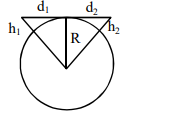# A transmitting antenna at top of a tower has a height of

Question:

A transmitting antenna at top of a tower has a height of $50 \mathrm{~m}$ and the height of receiving antenna is $80 \mathrm{~m}$. What is range of communication for Line of Sight (LoS) mode ?

[use radius of earth $=6400 \mathrm{~km}$ ]

1. $45.5 \mathrm{~km}$

2. $80.2 \mathrm{~km}$

3. $144.1 \mathrm{~km}$

4. $57.28 \mathrm{~km}$

Correct Option: , 4

Solution:$\mathrm{d}_{\mathrm{t}}=\sqrt{2 \mathrm{Rh}_{1}}+\sqrt{2 \mathrm{Rh}_{2}}$

$=\sqrt{2 \mathrm{R}}\left(\sqrt{\mathrm{h}_{1}}+\sqrt{\mathrm{h}_{2}}\right)$

$=\left(2 \times 6400 \times 10^{3}\right)^{1 / 2}(\sqrt{50}+\sqrt{80})$

$=3578(7.07+8.94)$

$=57.28 \mathrm{Km}$